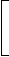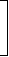# Aptitude - Banker's Discount - Discussion

Discussion Forum : Banker's Discount - General Questions (Q.No. 1)
1.
The banker's discount on a bill due 4 months hence at 15% is Rs. 420. The true discount is:
Rs. 400
Rs. 360
Rs. 480
Rs. 320
Explanation:
T.D.
 = B.D. x 100 100 + (R x T)
= Rs.420 x 100100 +15 x 13
 = Rs.420 x 100105
= Rs. 400.
Discussion:
69 comments Page 1 of 7.

Namaste prakash said:   5 months ago
15 percent per annum.
Hence;
15 x 4/12 = 15x 1/3= 5;
100 + 5 = 105.
(1)

Shikha said:   1 year ago
Please share with formula and solution.
(2)

Manjunath said:   4 years ago
@All.

Explanation of what is BG(Bankers gain), TD(True discount), BD(Bankers discount)
Example:

A Sales BIKE worth of 12000 to B with agreement. In agreement B took 3 month time for payment. Due to some problem A needs money after one month. While that time B Gave that agreement to bank and borrow money.

A simply not give 3 months of time he put some interest say 10% per anum.
Hence - use PTR/100 formula we get interest -300,
One month is over so bank pay 12100 to A,
TD = 12300 - 12100= 200.
Bank also needs some profit so bankers put interest on total amount {12000+300(interest)} =12300.

Let's calculate interest using - PTR/100.

While calculating interest take T - 2 month because already one month is over.
After calculation we get - 205 rupees is BANK DISCOUNT.
Bank Gain = B.D - T.D = 205 - 200 = 5 rupees.
(2)

Jubair said:   5 years ago
I think it will be too much easy if we take this as present value and future value.
Here banker's discount on a bill due 4 months hence at 15 % is RS 420, this 420 is the future value which we will get after 4 months. But what is the present value of that 420 is the true discount and the required answer.

So we can solve it solve it in Pv formula where,

PV = FV /(1+rn).
= 420 /1+ (15/100 * 4/12),
= 420 * 100/105,
= 400.

we can also take it as amount which is 420.
so P = 420 * 100/ (n*r)+100,
= 420 * 100/105,
= 400.
(3)

Lavanya Katturoju said:   5 years ago
How 100 + (15 * 1/3) is 105? Plase explain.
(2)

Skiezz said:   5 years ago
BD=(A*R*T)/100.
TD=(A*R*T)/100+(R*T),
TD=BD*100/100+(R*T),
{BD*100= (A*R*T)/100*100}.

That's why BD*100=A*R*T.

Roshu said:   5 years ago
Actually BD=amt*rate*time/100.

TD=amt*rate*time/100+(rate*time).
BD *100=amt*rate*time.
we are substituting the amt*rate*time in TD with BD*100 from the above step,
so TD=BD*100/100+(rate*time).
so TD=420*100/100+(15*1/3).
We write 4 months as 1/3 because the time should be expressed in terms of years,so 4/12=1/3.
Thus we get 420*100/105=400.

Hope this helps.
(1)

Yasmeen said:   5 years ago
What about 105 how it comes?

Yuga said:   6 years ago
Can anyone explain the problem, please?

Nanthini said:   6 years ago